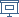FLOC 2018: FEDERATED LOGIC CONFERENCE 2018
PC ON SATURDAY, JULY 7TH
Days:
next day
all days

View: session overviewtalk overviewside by side with other conferences

10:30-11:00Coffee Break
12:30-14:00Lunch Break
14:00-15:30 Session 28K
Location: Maths L6
14:00
Space Proof Complexity beyond Resolution

ABSTRACT. Starting from results and techniques to prove lower and upper bounds for space complexity of resolution refutations, we will show some results about the state of the art of space bounds for the complexity of proofs in proof systems beyond resolution, like polynomial calculus, cutting planes and Frege systems, underlying open problems and  their importance.

15:00
Branching Program Complexity of Canonical Search Problems and Proof Complexity of FormulasABSTRACT. It is well-known that there is an equivalence between ordered resolution and ordered binary decision diagrams (OBDD); i.e., for any unsatisfiable formula phi, the size of the smallest ordered resolution refutation of phi equal to the size of the smallest OBDD for the canonical search problem corresponding to phi. But there is no such equivalence between resolution and branching programs (BP).

In this project, we study different proof systems equivalent to classes of branching programs between BP and OBDD. These proof systems are similar to roABP-IPS, an algebraic proof system defined by Forbes et al. and based on the ideal proof system introduced by Grochow and Pitassi.

We show that proof systems equivalent to b-OBDD are not comparable with resolution and cutting planes (for b > 1). We also prove exponential lower bounds for these proof systems on Tseitin formulas. Additionally, we show that proof systems equivalent to (1,+b)-BP are strictly stronger than regular resolution for b > 0; moreover, resolution does not p-simulate (1, +b)-BP for b > 5.

15:30-16:00Coffee Break
16:00-18:10 Session 31M
Location: Maths L6
16:00
17:00
Resolution and the binary encoding of combinatorial principles

ABSTRACT. We give lower bounds in Resolution-with-bounded-conjunction, Res\$(s)\$, for families of contradictions where witnesses are given in the unusual binary encodings. The two families we focus on are the \$k\$-Clique Formulas and those associated with the (weak) Pigeonhole Principle. If one could give lower bounds in Res\$(\log\$) for such families under the binary encoding, then these would translate to lower bounds for the more typical unary encoding in Resolution, Res\$(1)\$. Such a lower bound is not possible for certain very weak Pigeonhole Principles, but might be dreamt of for the \$k\$-Clique Formulas.

17:20
Resolution with Counting: Different Moduli and Tree-Like Lower Bounds
SPEAKER: Fedor Part

ABSTRACT. We study the complexity of resolution extended with the ability to count over different characteristics and rings. These systems capture integer and moduli counting, and in particular admit short tree-like refutations for insolvable sets of linear equations. For this purpose, we consider the system ResLin(R), in which proof-lines are disjunction of linear equations over a ring R.(We focus on the case where the variables are Boolean, i.e., we add the Boolean axioms (x=0)\/(x=1), for all variables x.) Extending the work of Itsykson and Sokolov we obtain new lower bounds and separations, as follows:

Finite fields:

Exponential-size lower bounds for tree-like ResLin(Fp) refutations of Tseitin mod q formulas, for every pair of distinct primes p,q. As a corollary we obtain an exponential-size separation between tree-like ResLin(Fp) and tree-like ResLin(Fq).

Exponential-size lower bounds for tree-like ResLin(Fp) refutations of random k-CNF formulas, for every prime p and constant k.

Exponential-size lower bounds for tree-like ResLin(F) refutations of the pigeonhole principle, for every field F.

All the above hard instances are encoded as CNF formulas. The lower bounds are proved using extensions and modifications of the Prover-Delayer game technique and size-width relations.

Characteristic zero fields:

Separation of tree-like ResLin(F) and (dag-like) ResLin(F), for characteristic zero fields F. The separating instances are the pigeonhole principle and the Subset Sum principle. The latter is the formula a1 x1 +... +an xn = b, for some b not in the image of the linear form. The lower bound for the Subset Sum principle employs the notion of immunity from Alekhnovich and Razborov.

17:40
Proof systems for #SAT based on restricted circuits

ABSTRACT. In this work, we extend a well-known connection between linear resolution refutation and read-once branching program by constructing proof systems based on syntactically restricted circuits studied in the field of Knowledge Compilation. While our approach only yield proof systems that are weaker than resolution, they may be used to define proof systems for problems such as #SAT or MaxSAT. This is a work in progress.

19:45-22:00 Workshops dinner at Balliol College

Workshops dinner at Balliol College. Drinks reception from 7.45pm, to be seated by 8:15 (pre-booking via FLoC registration system required; guests welcome).

Location: Balliol College